# Non Verbal Reasoning - Dot Situation

Exercise : Dot Situation - Section 1
Directions to Solve

From amongst the figures marked (1), (2), (3) and (4), select the figure which satisfies the same conditions of placement of the dots as in figure (X).

1.

Select the figure which satisfies the same conditions of placement of the dots as in Figure-X.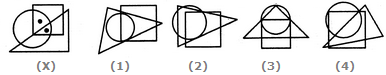1
2
3
4
Explanation:
In fig. (X), one of the dots lies in the region common to the circle and the square only and the other dot lies in the region common to all the three figures -the circle, the square and the triangle. In each of the alternatives (1), (2) and (3), there is no region common to the square and the circle only. Only fig. (4) consists of both the types of regions.

2.

Select the figure which satisfies the same conditions of placement of the dots as in Figure-X.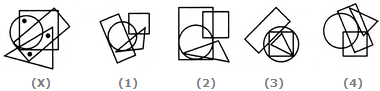1
2
3
4
Explanation:
In fig. (X), one of the dots lies in the region common to the circle and the square only, another dot lies in the region common to the square, the triangle and the rectangle only and the third dot lies in the region common to the triangle and the rectangle only. In each of the figures (1), (2) and (3) there is no region common to the square, the triangle and the rectangle only. Only fig. (4) consists of all the three types of regions.

3.

Select the figure which satisfies the same conditions of placement of the dots as in Figure-X.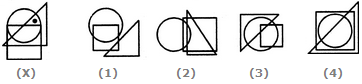1
2
3
4
Explanation:
In fig. (X), the dot is contained in the region common to the triangle and the circle only. Out of the four alternatives, only fig. (3) contains a region common to the triangle and the circle only.

4.

Select the figure which satisfies the same conditions of placement of the dots as in Figure-X.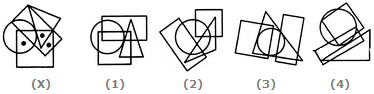1
2
3
4
Explanation:
In fig. (X), one of the dots lies in the region common to the circle and the square only, another dot lies in the region common to the square, the triangle and the rectangle only and the third dot lies in the region common to the square and the rectangle only. In fig. (2) there is no region common to the square and the rectangle only, in fig. (3) there is no region common to the circle and the square only and in fig. (4) there is no region common to the square, the triangle and the rectangle only. Only fig. (1) consists of all the three types of regions.

5.

Select the figure which satisfies the same conditions of placement of the dots as in Figure-X.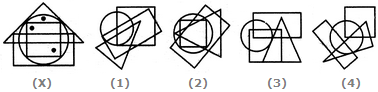1
2
3
4